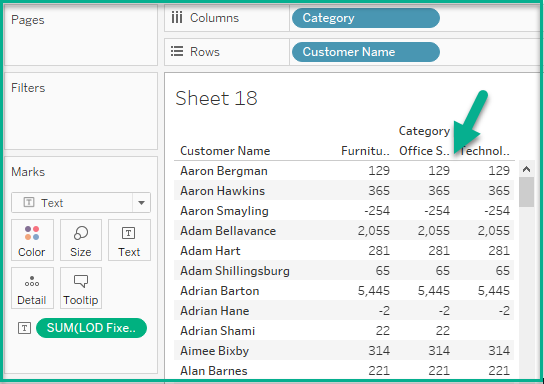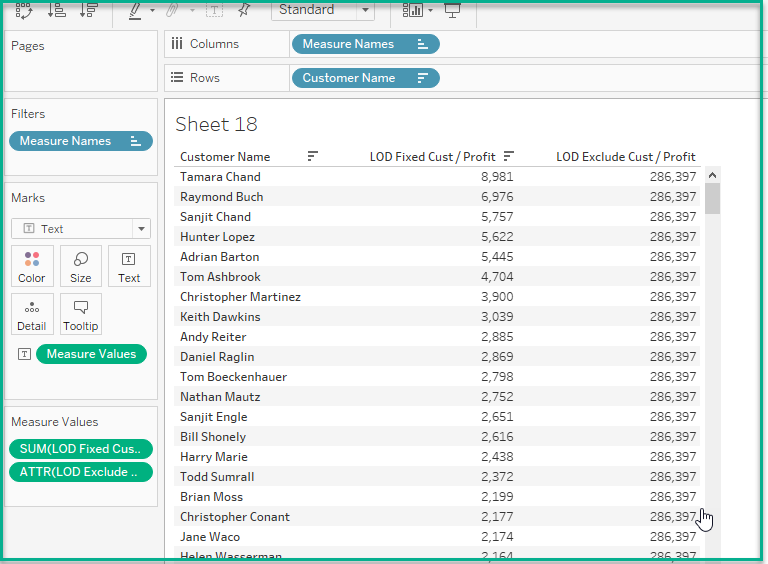# What is a LOD expression?

Have you heard of Level of Detail Expressions for Tableau? Why would someone want to use this type of calculation?

There are several kinds of LOD expressions;

• Include

• Exclude

• Fixed

You would use LOD expressions if you want to control how granular your calculations are.

### INCLUDE Example

Suppose I wanted to figure out what the Average Profit was for each Customer for the Category metric using the Sample Super-store data.

I created a simple bar chart showing the Average of Profit by the Category.I then created the following LOD expression;

LOD Include Cust / Profit

{ Include [Customer Name] : SUM([Profit])}

I placed this on my Rows shelf before the Average of Profit and changed the Measure to reflect Average instead of Sum.

As you can see in my Tooltip, the Average Profit for Furniture is higher than the straight Average. This is because Tableau is performing the calculation at the Customer Name level.Here is the overall view of the chart;### FIXED Example

So what happens when you do a Fixed LOD expression? When you use the term Fixed in your calculation it will calculate based on your expression. The measures in the view will not be considered in this.

If we go back to the our dashboard and create the following calculated field;

LOD Fixed Cust / Profit

{ FIXED [Customer Name] : SUM([Profit])}

If we place this on our Rows shelf, we notice the values jump considerably;What happened? Our Fixed LOD expression is only looking at the Profit by Customer name. As you can see in the print screen below the amount stays the same regardless of the Category.### EXCLUDE Example

Exclude LOD expressions omit the view from the level of detail. In our example it omits the Customer Name granularity from the calculation.

Why would you use this? These expressions are good for calculating the percent of the total.

If we go back to our example, we can find out which customers are the most profitable using the Exclude expression along with another calculated field. We could use these for our marketing efforts.

Here is our calculated field;

LOD Exclude Cust / Profit

{ Exclude [Customer Name] : SUM([Profit])}

Here is the view I created using this expression;I can create another calculated field to see what percent of the total these top customers are.

LOD Percent of Total

SUM([Profit])/SUM([LOD Exclude Cust / Profit])

which is the same as using this;

SUM([Profit])/SUM({Exclude [Customer Name] : SUM(Profit)})

If we add this calculation to the view and format it as a percentage, we can see that our top profitable customer makes up 3% of our total profit.